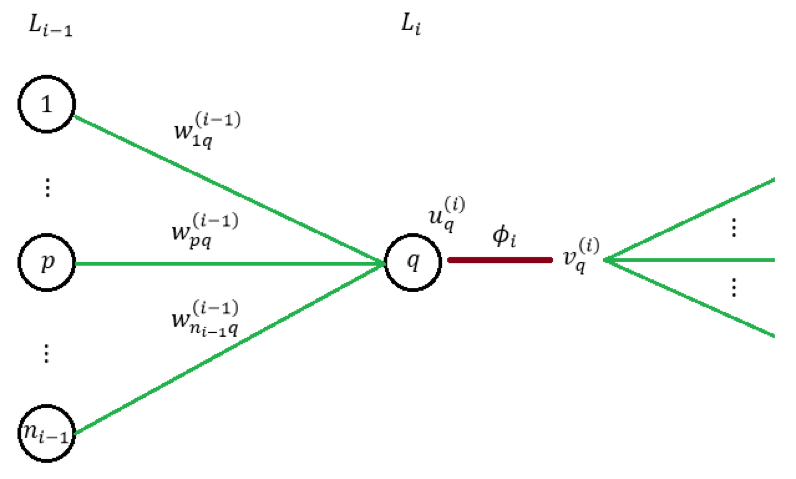# BP 算法的推导

• 梯度是向量函数$f$在某点$x$上升最快的方向、其数学定义为 它是向量函数$f$对 n 个分量的偏导组成的向量。需要指出的是，我个人的习惯是在推导向量函数的梯度时，先把它分拆成单个的函数进行普通函数的求偏导计算、最后再把它们合成梯度。后文的推导也会采取这种形式
• BP 算法的初始输入是真实的类别向量$y$
• 我们的目标是让模型的输出尽可能拟合$y$。为此我们会定义：
• 预测函数$f(x)$，它是一个向量函数、会根据输入矩阵$x$输出预测向量$v^{\left( m \right)}$
• 损失函数$L^{*}\left( x \right) \triangleq L\left( y,v^{\left( m \right)} \right) = L\left( y,f\left( x \right) \right)$，它是一个标量函数、其函数值能反映$v^{\left( m \right)}$$y$的差异；差异越大、$L^{*}\left( x \right) = L\left( y,v^{\left( m \right)} \right)$的值就越大• 当前 Layer 是 CostLayer、也就是说最后一层，此时有： 相当长的式子、里面涉及到的定义也挺多，不过其实每一步的本质都只是链式法则而已。注意最后出现了$\phi_{m}^{'}\left( u_{q}^{\left( m \right)} \right)$一项，它其实是输出层激活函数对应的导函数
• 当前 Layer 不是最后一层时，同样由链式法则可知（注意：该层的每个神经元对下一层所有神经元都会有影响） 其中 即为下一层传播回来的局部梯度；且由于 从而可知

• 对 CostLayer 而言、有
• 对其余 Layer 而言、有

# Softmax$+$log-likelihood 组合

• 假设输入为$x$、输出为$y \in c_{k}$
• 假设模型在 Softmax 之前的输出为
• 假设模型的 Softmax 接受的输入为
• 假设模型在 Softmax 之后的输出为 其中
• 假设模型的损失为 log-likelihood：